Engineering ToolBox - Resources, Tools and Basic Information for Engineering and Design of Technical Applications!

# Pulleys

## Pulleys, blocks and tackles.

A pulley is a device that can increase the magnitude of an effort force

### Without Pulley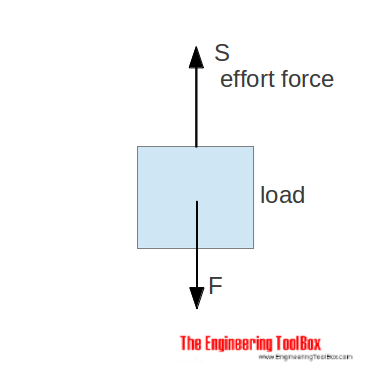With no pulley - the effort force is similar to the load - in opposite direction.

S = F                                  (1)

where

S = effort force (N, lb)

### Single Pulley

#### Fixed Pulley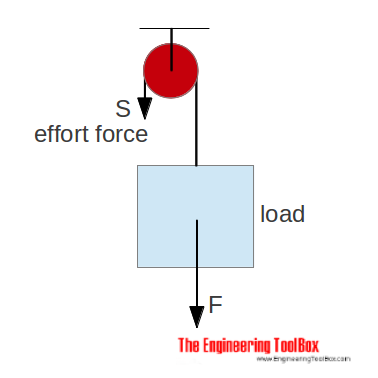With a single fixed pulley the effort force is similar (or more due to efficiency loss) to the load.

S = F                                (2)

The advantage with the single fixed pulley is that the direction of force is changed - it is possible to pull down instead of lifting up.

#### Movable Pulley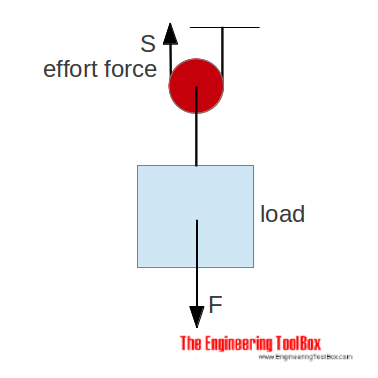With a single moveable pulley the effort force is half (or more due to efficiency loss) of the load.

S = 1/2 F                                (3)

### Combined Pulleys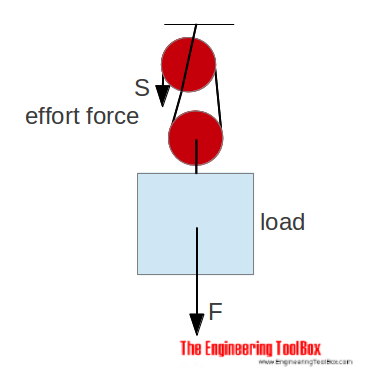With a combined moveable pulley as above - the effort force is half (or more due to efficiency loss) of the load.

S = 1/2 F                               (4)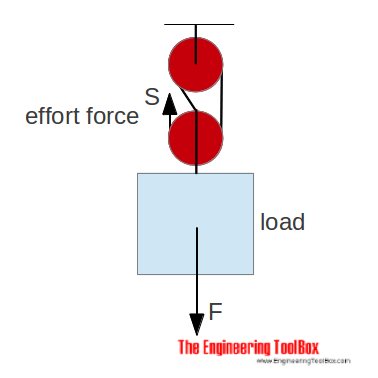With two pulleys and the ropes as above - the effort force is 1/3 (or more due to efficiency) of the load.

S = 1/3 F                             (5)

### General Equation for Blocks and Tackles

The general effort force equation for a block or tackle to raise or pull a load can be expressed as

S = F / (μ n)

= (m g) / (μ n)                                (6)

where

S = effort force (N, lb)

F = load (often weight) (N, lb)

m = mass (kg, slugs) (when lifting a mass)

g = constant of gravitation (9.81 m/s2, 32.17405 ft/s2) (when lifting a mass)

μ = mechanical efficiency of the system (equal to one for an ideal friction-less system, a fraction less than one for real-world systems with energy losses due to friction)

n = number of ropes between the sets of pulleys

### Block, Tackle or Pulley Calculator

The calculator below can be used to calculate effort force in block, tackle or pulley construction. The calculator can be used for metric and imperial units as long as the use of units are consistent.

F - load (N, kg, lb)

μ - mechanical efficiency of the system

n - number of ropes between the sets of pulleys

Note that kg is the SI units for mass - more about mass and weight!

### Example - Pulley and Effort Force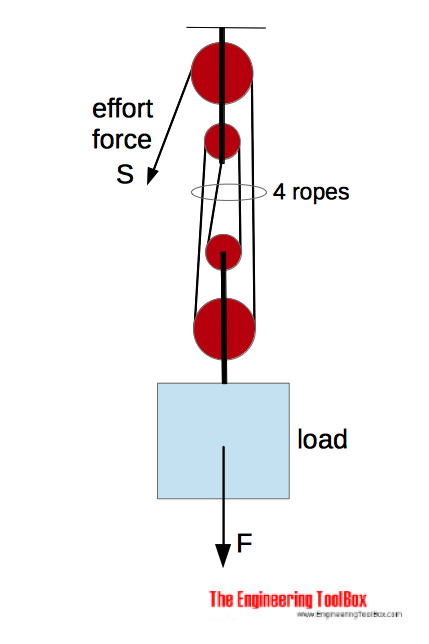The effort force for a pulley with 4 ropes, friction loss μ = 0.8, and load 100 kg can be calculated as

S = (100 kg) (9.81 m/s2) / ((0.8) (4))

= 307 N

## Related Topics

• Dynamics - Motion - velocity and acceleration, forces and torque.
• Mechanics - Forces, acceleration, displacement, vectors, motion, momentum, energy of objects and more.

## Engineering ToolBox - SketchUp Extension - Online 3D modeling!

Add standard and customized parametric components - like flange beams, lumbers, piping, stairs and more - to your Sketchup model with the Engineering ToolBox - SketchUp Extension - enabled for use with the amazing, fun and free SketchUp Make and SketchUp Pro .Add the Engineering ToolBox extension to your SketchUp from the SketchUp Pro Sketchup Extension Warehouse!

Translate

## Privacy

We don't collect information from our users. Only emails and answers are saved in our archive. Cookies are only used in the browser to improve user experience.

Some of our calculators and applications let you save application data to your local computer. These applications will - due to browser restrictions - send data between your browser and our server. We don't save this data.

## Citation

• Engineering ToolBox, (2007). Pulleys. [online] Available at: https://www.engineeringtoolbox.com/pulleys-d_1297.html [Accessed Day Mo. Year].

Modify access date.

. .

#### Scientific Online Calculator6 24